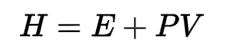Request a Tool

Enthalpy Calculator

Enthalphy is a measure of the total energy of a thermodynamic system

Enthalphy
0

Formula• H = Enthalpy
• E = Internal energy
• P = Pressure
• V = Volume

Defination / Uses

The total energy of a thermodynamic system is measured by enthalphy. It contains both the internal energy required to build a system and the energy required to make room for it by displacing its environment and establishing its volume and pressure.

Uses

The Enthalpy Calculator is used to calculate heat and mass transfer in a closed thermodynamic system under constant pressure during a nonlinear static operation (isobaric process).

The Enthalpy Calculator is used to calculate heat and mass transfer in a closed thermodynamic system under constant pressure during a nonlinear static operation (isobaric process). The Free Enthalpy Calculator is useful for calculating the enthalpy change of a reaction. The reaction scheme and the enthalpy formula are two easy ways for calculating the enthalpy change. To obtain a change in enthalpy value, students must choose one technique to calculate the enthalpy, provide the appropriate input details. Use the upper given formula for manual calculations. No sign-up, registration OR captcha is required to use this tool.